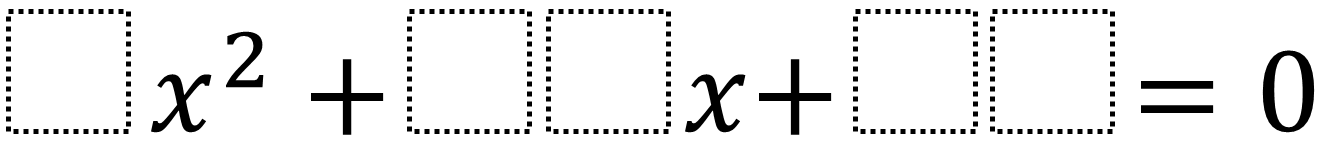Home > High School: Algebra > Factoring Quadratics 2 – Integer Solutions

# Factoring Quadratics 2 – Integer Solutions

Directions: Using the digits 0 to 9 at most one time each, fill in the boxes to so that the solutions are integers. Note that you can use a 05 to make a 5.### Hint

How could you find the equation if you already knew the solutions?

There are many answers including 3x^2 + 12x + 09 which has solutions of -1 and -3.

Source: Daniel Luevanos

## Solving Equations In Two Variables

Directions: Using the digits 1 to 9 at most one time each, fill in the …

1.2.3.